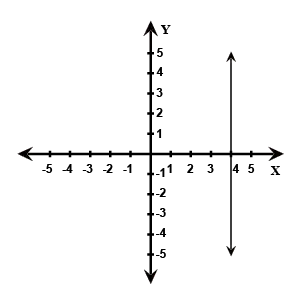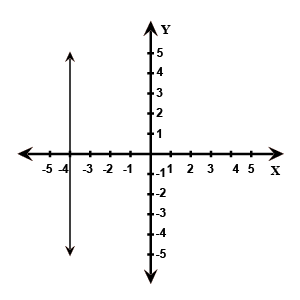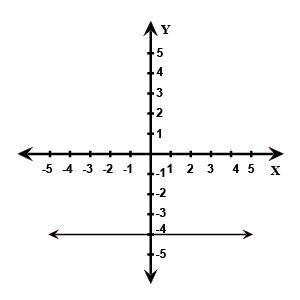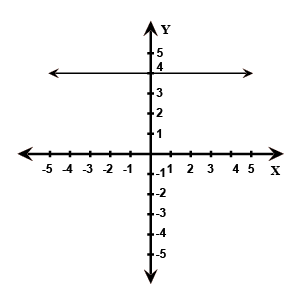Find the graph that represents the equation y=4 correctly.

# Find the graph that represents the equation ${y}{=}{4}$ correctly.

1. A2. B3. C4. DFill Out the Form for Expert Academic Guidance!l

+91

Live ClassesBooksTest SeriesSelf Learning

Verify OTP Code (required)

### Solution:

Given the equation is, ${y}{=}{4}$.
Since for every value of x we get only one value of y. i.e., ${y}{=}{4}$.
Therefore, the given equation is parallel to the x-axis and the distance is 4 above the x-axis.Therefore, option 4) is correct.

## Related content

 Area of Square Area of Isosceles Triangle Pythagoras Theorem Triangle Formula Perimeter of Triangle Formula Area Formulae Volume of Cone Formula Matrices and Determinants_mathematics Critical Points Solved Examples Type of relations_mathematics+91

Live ClassesBooksTest SeriesSelf Learning

Verify OTP Code (required)